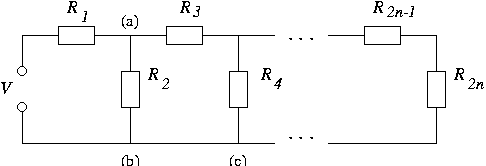EEE1004: Project 2. Equivalent Resistance Calculations

Consider the electrical circuit shown in the figure below:Such circuits are often called Ladder Networks.

This circuit contains 2n resistors connected in a ``series-parallel'' way. Every resistor whose number is odd (i.e., 1,3, ..., 2n-1) is connected in series with the group of resistors whose numbers are greater that its number. Those resistors whose numbers are even (i.e., 2,4, ..., 2n) are connected in parallel with the group of resistors whose numbers are greater. For example, R(1) is in series with the group between points (a) and (b), that is consisting of R(2),R(3), ..., R(2n). On the other hand, R(2) is in parallel with the group of resistors between points (a) and (c), that is R(3),R(4), ..., R(2n). Only the last two resistors, R(2n-1) and R(2n) are connected in series.

Design, implement and document a program that should perform the following:
• (Part 1) The program should calculate the overall equivalent resistance of the circuit connected to a voltage source V for a given set of values:
• Number of Resistors, and
• Individual Resistances (say, in Ohms) of Resistors R(1),R(2), ...,R(2n).

The program should request these values from the user, input them, and store the resistance values in an appropriate array before performing calculations.

Hint. Make use of the formulas for series and parallel interconnection of two resistors r(1) and r(2): Rseries = r(1) + r(2); Rparallel = (r(1) r(2)/(r(1)+r(2).
• (Part 2) In addition to the calculations performed for Part 1, the program should calculate the voltage that falls across and the current that flows through each resistor R(i) (V(i) and I(i), respectively), assuming that the value of the source voltage V is entered.
For part 2, the program should be able to read the parameters of the circuit from an input data file where the data is formatted in the following way:

24
6
100
200
100
200
100
100

i.e. the first line of the file contains the source voltage (24) in Volts, the second line the number of resistors in the circuit (6), and subsequent lines contain the individual resistances (in Ohms), one value per line.
The results should be written by the program into an output file containing a list of triplets (resistance R(i) in Ohms, voltage V(i) in Volts and current I(i) in Amps), formatted as follows:

100 12 .12
200 12 .06
etc.

i.e. one triplet per line, with the values separated by at least one space.

Note: In Part 2, your program is also expected to ask the user to select between two forms of data input (interactive, as in Part 1, or file) and provide the names of the input and output files.

Instructions for preparing your documentation are on: http://www.staff.ncl.ac.uk/alex.yakovlev/eee1004/course/proj-inst.reduced.html
09/11/07 A. Yakovlev